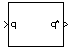# Quaternion Conjugate

Calculate conjugate of quaternion

• Library:
• Aerospace Blockset / Utilities / Math Operations

•## Description

The Quaternion Conjugate block calculates the conjugate for a given quaternion. Aerospace Blockset™ uses quaternions that are defined using the scalar-first convention. For more information on quaternion forms, see Algorithms

## Ports

### Input

expand all

Quaternions in the form of [q0, r0, ... , q1, r1, ... , q2, r2, ... , q3, r3, ...], specified as a quaternion or vector.

Data Types: `double` | `bus`

### Output

expand all

Quaternion conjugates in the form of [q0′, r0′, ... , q1′, r1′, ... , q2′, r2′, ... , q3′, r3′, ...], returned as a quaternion or vector.

Data Types: `double` | `bus`

## Algorithms

The quaternion has the form of

`$q={q}_{0}+i{q}_{1}+j{q}_{2}+k{q}_{3}.$`

The quaternion conjugate has the form of

`${q}^{\prime }={q}_{0}-i{q}_{1}-j{q}_{2}-k{q}_{3}.$`

 Stevens, Brian L., Frank L. Lewis. Aircraft Control and Simulation, Second Edition. Hoboken, NJ: Wiley–Interscience.

## Support